index: click on a letter A B C D E F G H I J K L M N O P Q R S T U V W X Y Z A to Z index index: subject areas numbers & symbols sets, logic, proofs geometry algebra trigonometry advanced algebra & pre-calculus calculus advanced topics probability & statistics real world applications multimedia entrieswww.mathwords.com about mathwords website feedback

Inverse of a Matrix
Matrix Inverse
Multiplicative Inverse of a Matrix

For a square matrix A, the inverse is written A-1. When A is multiplied by A-1 the result is the identity matrix I. Non-square matrices do not have inverses.

Note: Not all square matrices have inverses. A square matrix which has an inverse is called invertible or nonsingular, and a square matrix without an inverse is called noninvertible or singular.

AA-1 = A-1A = I

 Example: For matrix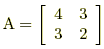, its inverse is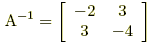since AA-1 =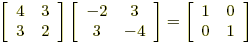and A-1A =.

Here are three ways to find the inverse of a matrix:

 1. Shortcut for 2x2 matrices For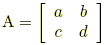, the inverse can be found using this formula: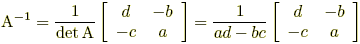Example:2. Augmented matrix method Use Gauss-Jordan elimination to transform [ A | I ] into [ I | A-1 ]. Example: The following steps result in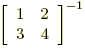.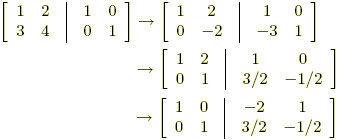so we see that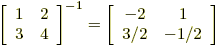. 3. Adjoint method A-1 =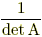(adjoint of A)   or   A-1 =(cofactor matrix of A)T Example: The following steps result in A-1 for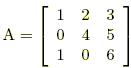. The cofactor matrix for A is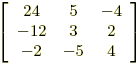, so the adjoint is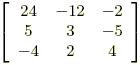. Since det A = 22, we get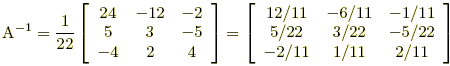.